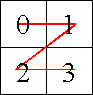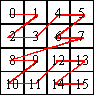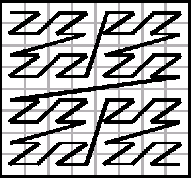### Problem Statement

A Z-curve is a path that traces through all the points in a two-dimensional square grid in such a way that the four quadrants are visited in order: first the upper left, then the upper right, then the lower left, and finally the lower right. If those quadrants contain more than 1 cell, these cells are visited recursively, in the same manner. A Z-curve of order N is a path through a 2^N by 2^N grid. A Z-curve of order 1 is a simple Z-shape through a 2-by-2 grid, as shown below.Here is a diagram of a Z-curve of order 2.Notice the order each quadrant was visited:

- upper left quadrant: 0, 1, 2, 3

- upper right quadrant: 4, 5, 6, 7

- lower left quadrant: 8, 9, 10, 11

- lower right quadrant: 12, 13, 14, 15

You will be given a value N and coordinates r and c denoting the row and the column in a 2^N by 2^N grid. Coordinates range from 0 to (2^N)-1 inclusive, with the upper left corner at coordinates (0,0). Assuming you start from the upper left corner and the points are traversed in the order specified by a Z-curve of order N, determine how many steps it takes to reach the point at coordinates (r,c).

### Definition

 Class: ZCurve Method: zValue Parameters: int, int, int Returns: int Method signature: int zValue(int N, int r, int c) (be sure your method is public)

### Constraints

-N is between 1 and 15, inclusive.
-r and c are between 0 and 2^N-1, inclusive.

### Examples

0)

 `2` `3` `1`
`Returns: 11`
1)

 `1` `0` `0`
`Returns: 0`
2)

 `3` `7` `7`
`Returns: 63`
 (7,7) is in the lower right corner of the grid and, as shown in the following diagram, it is the last point visited.3)

 `4` `7` `7`
`Returns: 63`
 The upper left quadrant of a Z-curve of order 4 is actually a Z-curve of order 3 and, because it is the first quadrant visited, the answer is the same as in the previous example.
4)

 `10` `511` `511`
`Returns: 262143`
5)

 `10` `512` `512`
`Returns: 786432`

#### Problem url:

http://www.topcoder.com/stat?c=problem_statement&pm=4808

#### Problem stats url:

http://www.topcoder.com/tc?module=ProblemDetail&rd=7999&pm=4808

supernova

#### Testers:

PabloGilberto , brett1479 , Olexiy

#### Problem categories:

Recursion, Simple Math